Antiferromagnetic Transition in the Hubbard Model

D. Stanford May 20, 2008

(Submitted as coursework for Physics 373, Stanford University, Spring 2008)

We demonstrate the existence, in mean field theory, of an antiferromagnetic transition in the transverse-field Hubbard Model and present the phase diagram.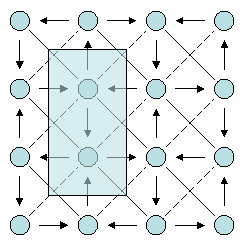Fig. 1: Our gauge choice for the problem. Unit cell shown shaded in blue.

The Model

The Hubbard model is a stylized description of fermions interacting via short ranged interactions. The lattice spacing (set equal to 1 in this report) provides a UV regulator, while the IR effects are regulated by replacing the Coulomb interaction by a delta function in space. Mathematically, the Hamiltonian is given by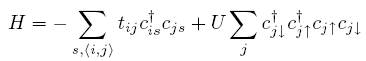We use the t elements described in Fig. 1. Lines with arrow represent n.n. hopping elements of strength t and phase &pi/4. Single lines represent diagonal hops, with strength t', and phase 0 (dashed) or &pi (solid). This seemingly arbitrary choice corresponds physically to introducing a unit of magnetic flux through each plaquette and exploiting gauge freedom to reduce the unit cell to two sites.

Mean-Field Solution

In the mean field approximation, we replace the quartic interaction by a quadratic one via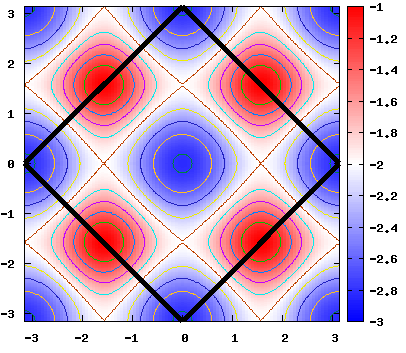Fig. 2: The energy dispersion for the transverse-field, U=0 Hubbard model, as a function of x and y momenta. The Brillouin zone can be chosen to be within the black diamond.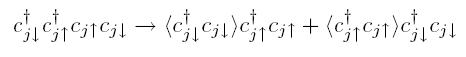Anticipating antiferromagnetism, we guess the mean field values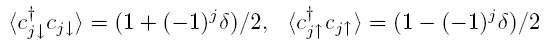where &delta is the antiferromagnetic order parameter. With this approximation in place, computing the unit cell Hamiltonian is straightforward: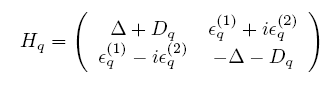where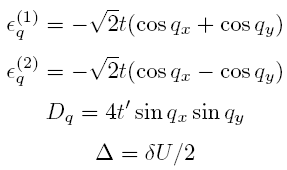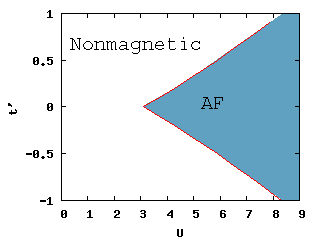Fig. 3:The phase diagram for our model, in mean field approximation. The code used to produce the coordinates of the boundary is here.

This Hamiltonian admits the energy eigenvalues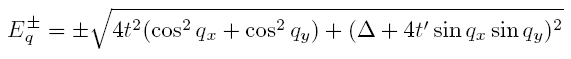The energy spectrum for U=0 is plotted over the Brillouin zone in Fig.1.

Antiferromagnetism

With the mean field hamiltonian in hand, we are in a position to determine the antiferromagnetic order parameter self consistently. To do so, we impose the constraint given by the usual "gap equation" which takes the form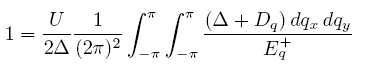This constraint gives &Delta(t,t',U), and thus &delta(t,t',U). Since the integral is convergent for any finite value of &Delta, there are values of U for which there is no self-consistent solution. Physically, the mean field version of our system exhibits a phase transition from nonmagnetic (no solution) to antiferromagnetic (solution exists) at a finite value of U. By evaluating the gap equation integral numerically, we can reconstruct the phase diagram for our model, as shown in Fig. 3. In particular, we find that antiferromagnetism does not emerge for values of U less than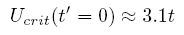See the next report for a discussion of the critical properties and emergence of a Goldstone mode near the phase boundary.

© 2007 D. Stanford. The author grants permission to copy, distribute and display this work in unaltered form, with attribution to the author, for noncommercial purposes only. All other rights, including commercial rights, are reserved to the author.